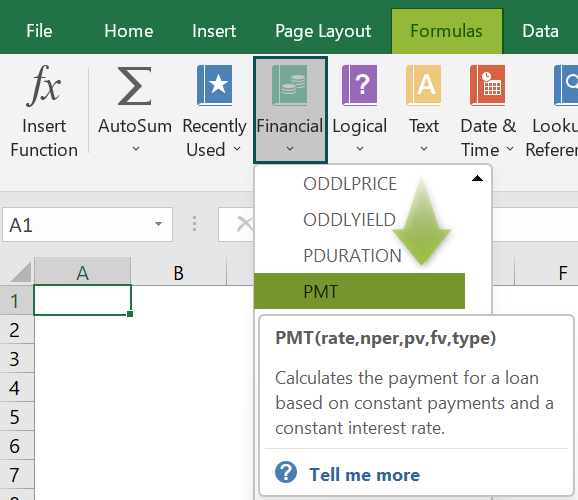# PPMT Function In ExcelArticle byExcelMojo Team## What Is PPMT Function In Excel?

The Periodic Payment or the PPMT function in Excel calculates the principal part/portion of the total loan amount. We can find the initial, final, or any periodic principal amount.

The PPMT Excel function is an inbuilt financial function, so we can insert the formula from the “Function Library” or enter it directly in the worksheet.

For example, we will use the PPMT Formula to calculate the principal amount of the loan amount of ‘\$20,000’, and the period is “12 years” at ‘10%’ of the interest rate. Select cell B5, enter the formula =PPMT(B4,1,B3,-B2), and press the “Enter” key. The result is ‘\$935.27’, as shown below.

###### Key Takeaways
• In a periodic loan payment, PMT is the total amount (interest amount + principal amount), IPMT is the interest portion of the total amount, and PPMT is the principal portion of the total amount. The PPMT function calculates the principal part of the total amount.
• The input rate has to be consistent. If the payments are made quarterly, it will convert the annual interest rate into the quarterly rate (rate%/4), and the period number has to be converted from years to quarters (=per*4).
• The present value of the loan amount is entered as negative because it is the amount that gets deducted periodically.

### PPMT() Excel Formula

The syntax of the PPMT Excel formula is,

The arguments of the PPMT Excel formula are,

• rate: It is a mandatory argument. It is the rate of interest on the loan.
• per: It is a mandatory argument. It is the total maturity date.
• nper: It is a mandatory argument. It is the total payment period.
• pv: It is a mandatory argument. It is the present value of the investment.
• fv: It is a mandatory argument. It is the future value of the investment.
• type: It is a mandatory argument. It specifies whether the payment is made at the start or the end of the period.

### How To Use PPMT Excel Function?

We can use the PPMT excel function 2 ways, namely,

1. Access from the Excel ribbon
2. Enter in the worksheet manually

#### Method #1 – Access from the Excel ribbon

Choose an empty cell for the output → select the “Formulas” tab → go to the “Function Library” group → click the “Financial” option drop-down → select the “PPMT” function, as shown below.

The “Function Arguments” window appears. Enter the arguments in the “Rate”, “Per”, “Nper”, “Pv”, “Fv”, and “Type” fields → click “OK”, as shown below.

#### Method #2 – Enter in the worksheet manually

1. Select an empty cell for the output.
2. Type =PPMT( in the selected cell. [Alternatively, type =P or =PP and double-click the PPMT function in Excel from the list of suggestions shown by Excel.]
3. Enter the arguments as cell values or cell references and close the brackets.
4. Press the “Enter” key.

Let us take a basic example to understand this funciton.

Mr. Raj Mohan has taken a loan of \$5,00,000 for five years, and the interest rate is 4%. We will calculate his monthly PPMT using PPMT function.

In the table, the data is,

• Column A contains a Description.
• Column B contains Amount.

The steps to calculate the PPMT using PPMT function in Excel are,

1. Select cell B7, and enter the formula =PPMT(B4/12, i.e., the rate of interest value.2. Enter the value of ‘per’ as “1”, i.e., the period value. The formula now is =PPMT(B4/12,1,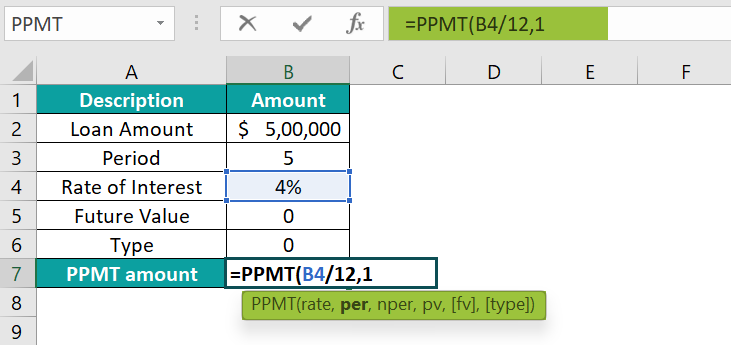3. Enter the value of ‘nper’ as “B312”, i.e., the monthly period value. The formula now is =PPMT(B4/12,1,B312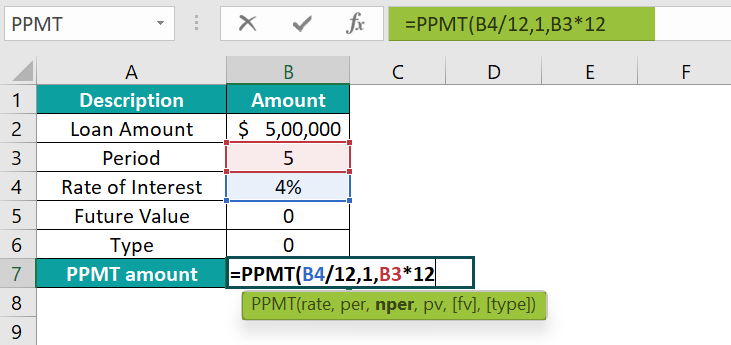4. Enter the value of ‘pv’ as “-B2”, i.e., the present value. The formula now is =PPMT(B4/12,1,B3*12,-B2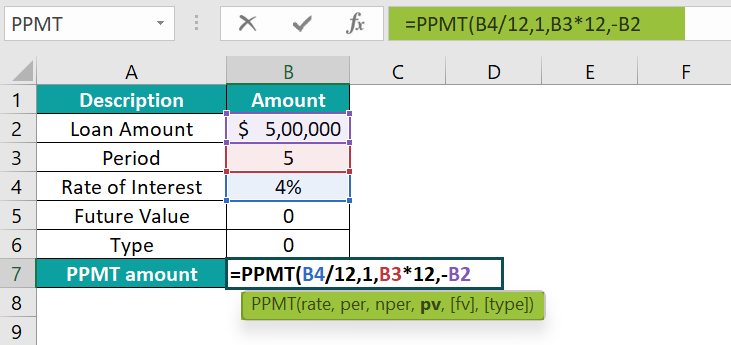5. Enter the value of ‘fv’ as “B5”, i.e., the future value. The formula now is =PPMT(B4/12,1,B3*12,-B2,B5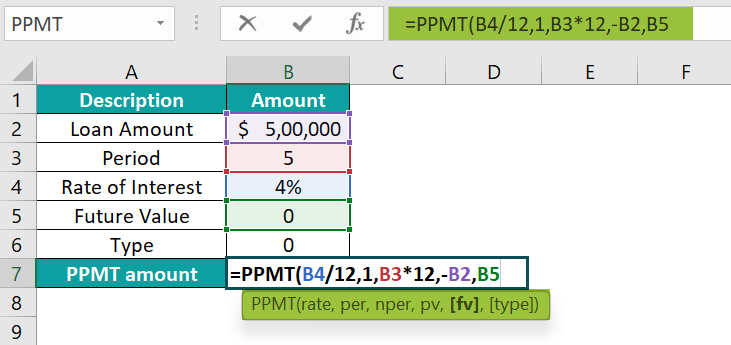6. Enter the value of ‘type’ as “0”, i.e., the payment type, and close the brackets. The complete formula is =PPMT(B4/12,1,B3*12,-B2,B5,0)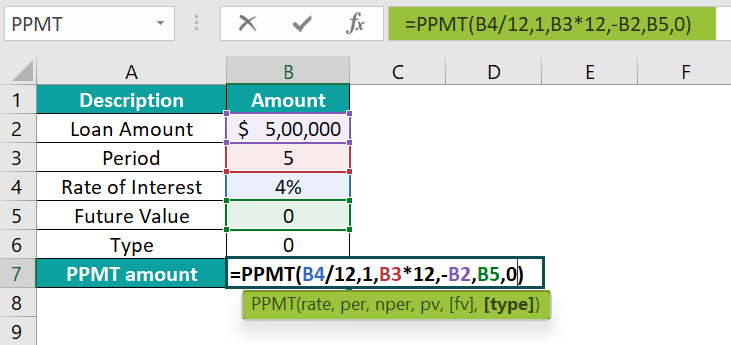7. Press the “Enter” key.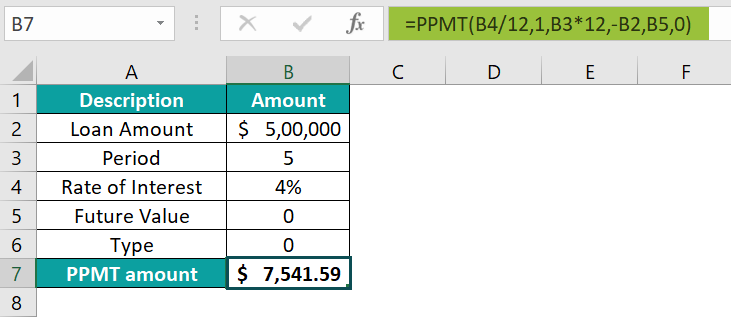The result is “\$7,541.59”, as shown above.

### Examples

We will understand some advanced scenarios using the PPMT function in Excel examples.

#### Example #1

Mrs. Lorence has borrowed \$80,000 for two years, and the interest rate is 15%. We will calculate his quarterly PPMT using the PPMT function in Excel.

In the table, the data is,

• Column A contains a Description.
• Column B contains Amount.

The procedure to calculate the PPMT using the PPMT function is:

Select cell B7, enter the formula =PPMT(B4/4,1,B3*4,-B2,B5,0), press the “Enter” key.

[Note: ‘rate’ as “B4/4”, ‘per’ as “1”, ‘nper’ as “B3*4” [since it is the quarterly period value], ‘pv’ as “-B2”, ‘fv’ as “B5”, and ‘type’ as “0”.]

The result is “\$8,759.87”, as shown above.

#### Example #2

XYZ company has taken a loan of \$25,00,000 for five years; the interest rate is 14%. We will calculate the company’s weekly PPMT using the PPMT function in Excel.

In the table, the data is,

• Column A contains a Description.
• Column B contains Amount.

The procedure to calculate the PPMT is:

Select cell B7, enter the formula =PPMT(B4/52,1,B3*52,-B2,B5,0),press the “Enter” key.

[Note: ‘rate’ as “B4/52”, ‘per’ as “1”, ‘nper’ as “B3*52” [since it is the weekly period value], ‘pv’ as “-B2”, ‘fv’ as “B5”, and ‘type’ as “0”.]

The result is “\$6,652.88”, as shown above.

#### Example #3

Ms. Kathrine has taken a loan of \$5,00,000 for five years, and the interest rate is 4%. We will calculate her semi-annual PPMT using the PPMT function in Excel.

In the table, the data is,

• Column A contains a Description.
• Column B contains Amount.

The procedure to calculate the PPMT using the PPMT Excel Function is,

Select cell B7, enter the formula =PPMT(B4/2,1,B3*2,-B2,B5,0),press the “Enter” key.

[Note: ‘rate’ as “B4/2”, ‘per’ as “1”, ‘nper’ as “B3*2” [since it is the semi-annual period value], ‘pv’ as “-B2”, ‘fv’ as “B5”, and ‘type’ as “0”.]

The result is “\$16,658.19”, as shown above.

### Important Things To Note

• The “#VALUE!” error occurs when:
• The values in the PPMT formula are non-numeric.
• The cell reference is incorrect.
• We don’t divide the interest based on annual, semi-annual, quarterly, monthly, or weekly period payments.
• The “#NUM! error” occurs if the “per” value is less than 0 or greater than the “nper” value.
• The type argument value will be either 0 or 1 for the payment made at the end or beginning of the period, respectively. If no value is provided in the formula, by default, it will be taken as 0.

1. What does the PPMT function in Excel mean in Excel?

The PPMT function in Excel calculates the principal share of the given loan payment and payment period. The payment for the initial, final, or any period in between is evaluated using the PPMT function in Excel.

The syntax of the PPMT function in Excel is =PPMT(rate,per,nper,pv,[fv],[type])

2. How does Excel PPMT function in Excel work in Excel?

The PPMT function in Excel works in Excel as follows:

1. Select an empty cell for the output.
2. Type =PPMT( in the selected cell. [Alternatively, type =P or =PP and double-click the PPMT function in Excel from the list of suggestions shown by Excel.]
3. Enter the arguments as cell values or cell references and close the brackets.
4. Press the “Enter” key.

For example, we apply the PPMT function in Excel to calculate the principal amount of the loan amount of ‘\$1,00,000’, and the period is “5 years” at ‘3%’ of the interest rate.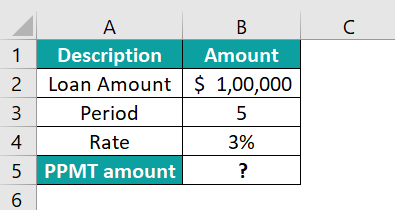Select cell B5, enter the formula =PPMT(B4/12,2,B3*12,-B2), and press the “Enter” key.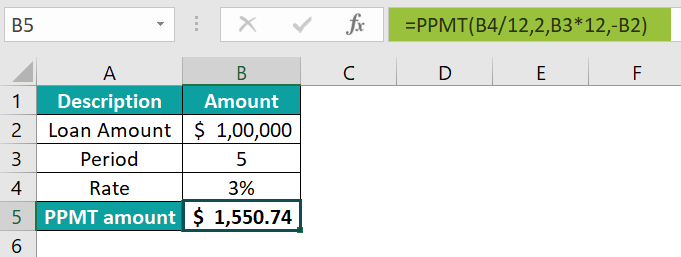The result is ‘\$1,550.74’, as shown above.

3. Where is the PPMT function in Excel in Excel?

The PPMT function in Excel is in the Formulas tab as follows:

Choose an empty cell for the output → select the “Formulas” tab → go to the “Function Library” group → click the “Financial” option drop-down → select the “PPMT” function, as shown below.